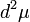# Number of conjugacy classes in a subgroup of finite index is bounded by index times number of conjugacy classes in the whole group

## Statement

### Statement in terms of number of conjugacy classes

Suppose$G$ is a group and$H$ is a subgroup of finite index. Suppose the number of conjugacy classes in$G$ is a finite number$c$ and the index of$H$ in$G$ is$d$. Then, the number of conjugacy classes in$H$ is at most$cd$.

### Statement in terms of commuting fraction

Suppose$G$ is a finite group and$H$ is a subgroup of finite index. Suppose the commuting fraction of$G$ is a finite number$\mu$ and the index of$H$ in$G$ is$d$. Then, the commuting fraction of$H$ is at most$d^2\mu$.